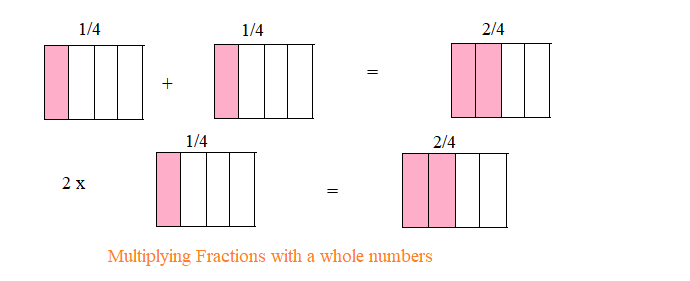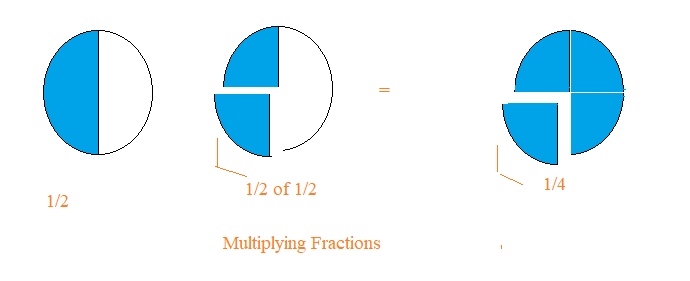# Multiplying Fractions | Multiply fractions with whole numbers

## Multiplication of a Fraction by a Whole Number

Multiplication of a fraction by a whole number can be understood by below example
Suppose we have 2 , 1/4 piece of chocolate. So total chocolate we have,
$\frac {1}{4} + \frac {1}{4} =\frac {2}{4}= \frac {1}{2}$
or
$\frac {1}{4} + \frac {1}{4} =2 \times \frac {1}{4}$
So,
$2 \times \frac {1}{4} =\frac {2}{4}= \frac {1}{2}$How to multiply fractions with whole numbers
Here are the steps to multiply fractions with whole numbers
• if we have mixed fraction in the multiplication, first convert into improper fraction
• Now multiply the whole number with the numerator of the fraction, keeping the denominator same
• We can simplify the fractions if needed and if the multiplication fraction results in improper fraction, you can convert into mixed fraction if required.

Solved Examples
Question 1. $3 \times \frac {1}{5}$
$3 \times \frac {1}{5} = \frac {3 \times 1}{5}= \frac {3}{5}$

Question 2. $2 \times \frac {4}{5}$
$2 \times \frac {4}{5} = \frac {2 \times 4}{5}=\frac {8}{5} = 1 \frac {3}{5}$

Question 3. $2 \times 2\frac {1}{5}$
$2 \times 2\frac {1}{5} = 2 \times \frac {11}{5}=\frac {22}{5} = 4 \frac {2}{5}$

Question 4. $2 \times \frac {1}{8}$
$2 \times \frac {1}{8} =\frac {2}{8} = \frac {1}{4}$

## Fraction as an operator 'of'

'of' represent multiplication .So 1/2 of 4 is represent $\frac {1}{2} \times 4 = 2$
Question 1. $\frac {1}{5} \; of \; 20$
$\frac {1}{5} \; of \; 20 = \frac {1}{5} \times 20= \frac {20}{5} = 4$

Question 2. $\frac {4}{5} \; of \; 10$
$\frac {4}{5} \; of \; 10= \frac {4}{5} \times 10=\frac {40}{5} = 8$

## Multiplication of a Fraction by a Fraction/Multiplying Fractions

Let us understand Multiplying Fractions through an example.
Suppose Ram have half (1/2) piece of pizza. And he want to equally share that with his Friend Suresh. So each of them would get 1/2 of 1/2 which can be written as
$\frac {1}{2} \; of \; \frac {1}{2} = \frac {1}{2} \times \frac {1}{2}$.

Now we can easily see that each of them get 1/4 piece of the whole pizza actually.So
$\frac {1}{2} \; of \; \frac {1}{2} = \frac {1}{2} \times \frac {1}{2} = \frac {1}{4}$.Here we can see that Multiplying fraction is simply acheived by
$Multiplying \; Fractions= \frac {products \; of \; numerator}{products \; of \; denominators}$
$\frac {1}{2} \times \frac {1}{2} = \frac { 1 \times 1 }{2 \times 2} = \frac {1}{4}$

$\frac {1}{3} \times \frac {1}{2} = \frac { 1 \times 1 }{3 \times 2} = \frac {1}{6}$
$\frac {2}{3} \times \frac {1}{2} = \frac { 2 \times 1 }{3 \times 2} = \frac {2}{6} = \frac {1}{3}$
$\frac {2}{3} \times \frac {3}{4} = \frac { 2 \times 3 }{3 \times 4} = \frac {6}{12} = \frac {1}{2}$
How to multiply fractions with fractions
Here are the steps for multiplying fractions
• if we have mixed fraction in the multiplication, first convert into improper fraction
• Now obtain the products of the numerator and denominators.
• We can simplify the fractions if needed and if the multiplication fraction results in improper fraction, you can convert into mixed fraction if required.
Solved Examples
Question 1. $\frac {3}{2} \times \frac {1}{5}$
$\frac {3}{2} \times \frac {1}{5} = \frac {3 \times 1}{2 \times 5}= \frac {3}{10}$

Question 2. $\frac {3}{7} \times \frac {4}{5}$
$\frac {3}{7} \times \frac {4}{5} = \frac {3 \times 4}{7 \times 5}=\frac {12}{35}$

Question 3. $\frac {3}{7} \times 2\frac {1}{5}$
$\frac {3}{7} \times 2\frac {1}{5} = \frac {3/7} \times \frac {11}{5}=\frac {33}{35}$

Question 4. $2\frac {1}{5} \times 1\frac {1}{8}$
$2\frac {1}{5} \times 1\frac {1}{8} =\frac {11}{5} \times \frac {9}{8} = \frac {99}{40} = 2 \frac {19}{40}$
Value of Product of fractions
a. when two proper fractions are multiplied, the product is less than each of the fractions
b. the product of two improper fractions is greater than each of the two fractions.
Checkout Multiplying Fractions calculator

### Quiz Time

Question 1 Which of these fraction is greatest?
A) $\frac {1}{2} \times \frac {1}{2}$
B)$\frac {1}{2} \times \frac {1}{3}$
C)$\frac {1}{3} \times \frac {1}{3}$
D)$\frac {1}{4} \times \frac {1}{2}$
Question 2 1/12 of 36?
A)$\frac {1}{48}$
B)$\frac {1}{3}$
C) 3
D)None of these
Question 3 The value of multiplication $\frac {1}{11} \times \frac {9}{11}$
A)$\frac {10}{3}$
B)$\frac {9}{121}$
C)$\frac {1}{9}$
D)$1\frac {1}{3}$
Question 4 The value of multiplication $3 \times \frac {2}{9}$
A)$\frac {2}{3}$
B) $\frac {3}{2}$
C) $\frac {2}{9}$
D) $\frac {3}{2}$
Question 5 The value of multiplication $1\frac {1}{3} \times 2\frac {2}{5}$?
A)3$\frac {2}{5}$
B)3$\frac {1}{5}$
C)$\frac {1}{15}$
D)$\frac {2}{5}$